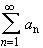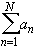#Interactive Real Analysis

Next | Previous | Glossary | Map

## 4.1. Series and Convergence

### Definition 4.1.2: Series, Partial Sums, and Convergence

Let {a n} be an infinite sequence.
1. The formal expressionis called an (infinite) series.
2. For N = 1, 2, 3, ... the expression S N =is called the N-th partial sum of the series.
3. If lim S N exists and is finite, the series is said to converge.
4. If lim S N does not exist or is infinite, the series is said to diverge.
Next | Previous | Glossary | Map